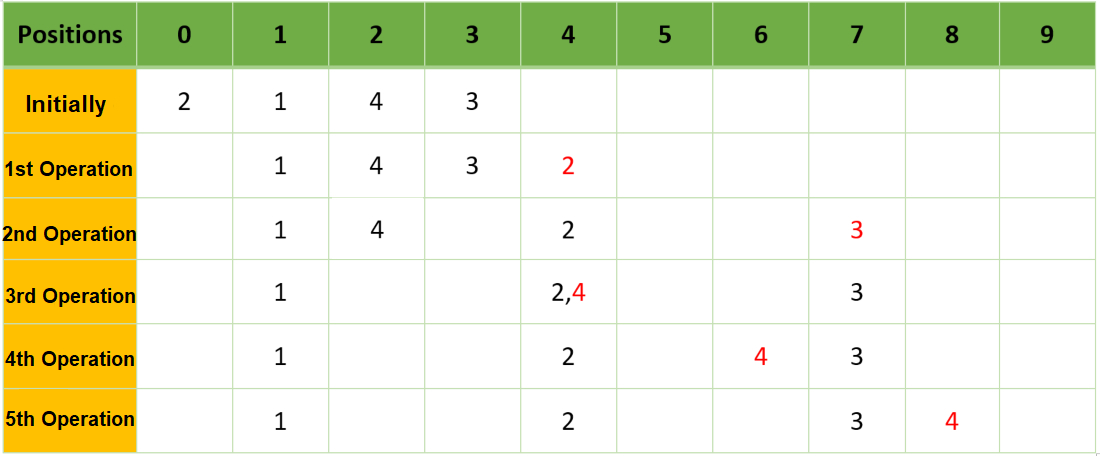# Sort elements of an array A[] placed on a number line by shifting i-th element to (i + B[i])th positions minimum number of times

• Difficulty Level : Easy
• Last Updated : 29 Jun, 2021

Given two arrays A[] and B[] consisting of N positive integers such that each array element A[i] is placed at the ith position on the number line, the task is to find the minimum number of operations required to sort the array elements arranged in the number line. In each operation any array element A[i] can move to the position (i + B[i])th on the number line. Two or more elements can come to the same number line.

Examples:

Input: A[] = {2, 1, 4, 3}, B[] = {4, 1, 2, 4}
Output: 5
Explanation:Initially array 2, 1, 4, 3 are placed on the number line at 0, 1, 2, 3 positions respectively.
Operation 1: Move arr(= 2) by move(= 4). Now the element are arranged as {1, 4, 3, 2} at indices on the number line is {1, 2, 3, 4} respectively.
Operation 2: Move arr(= 3) by move(= 4). Now the element are arranged as {1, 4, 2, 3} at indices on the number line is {1, 2, 4, 7} respectively.
Operation 3: Move arr(= 4) move(= 2). Now the element are arranged as {1, 2, 4, 3} at indices on the number line is {1, 4, 4, 7} respectively.
Operation 4: Move arr(= 4) move(= 2). Now the element are arranged as {1, 2, 3, 4} at indices on the number line is {1, 4, 6, 7} respectively.
Operation 5: In first operation move arr(= 2) i.e., 2 by move(= 4). Now the element are arranged as {1, 4, 3, 2} at indices on the number line is {1, 4, 7, 8} respectively.

Input: A[] = {1, 2, 3, 4}, B[] = {4, 1, 2, 4}
Output: 0

Approach: The given problem can be solved using the Greedy Approach by moving the greater element one by one to its next possible index and then find the minimum of all the operations required. Follow the steps below to solve the given problem:

• Initialize a 2D vectors, say arr[] such that each ith element represents the element, corresponding moves, and the current position as {arr[i], A[i], current_position}.
• Sort the array arr[] in ascending order.
• Initialize two variables, say cnt and f, and Mark count as 0 and flag as 1 to store the number of required operations.
• Iterate until F is not equal to 1 and perform the following steps:
• Update the value of F equals 0.
• For each element in vector arr[] and If the value of arr[i] is at least arr[i + 1], then increment the count by 1, f = 1 and the current position of the (i + 1)th element i.e., (arr[i + 1]) by arr[i + 1].
• After completing the above steps, print the value of count as the result.

Below is the implementation of the above approach:

## C++

 `// C++ program for the above approach` `#include ``using` `namespace` `std;` `// Function to find minimum number of``// operations required to sort N array``// elements placed on a number line``int` `minHits(``int` `arr[], ``int` `move[],``            ``int` `n)``{``    ``// Stores the value of``    ``// {A[i], B[i], current position}``    ``vector > V(n, vector<``int``>(3));` `    ``// Populate the current position``    ``// every elements``    ``for` `(``int` `i = 0; i < n; i++) {``        ``V[i] = { arr[i], move[i], i };``    ``}` `    ``// Sort the vector V``    ``sort(V.begin(), V.end());` `    ``// Stores the total number of``    ``// operations required``    ``int` `cnt = 0;``    ``int` `f = 1;` `    ``// Iterate until f equals 1``    ``while` `(f == 1) {` `        ``// Update f equals zero``        ``f = 0;` `        ``// Traverse through vector``        ``// and check for i and i+1``        ``for` `(``int` `i = 0; i < n - 1; i++) {` `            ``// If current position of``            ``// i is at least current``            ``// position of i+1``            ``if` `(V[i] >= V[i + 1]) {` `                ``// Increase the current``                ``// position of V[i+1]``                ``// by V[i+1]``                ``V[i + 1] += V[i + 1];` `                ``// Increment the count``                ``// of operations``                ``cnt++;` `                ``// Update the flag equals``                ``// to 1``                ``f = 1;` `                ``// Break the for loop``                ``break``;``            ``}``        ``}``    ``}` `    ``// Return the total operations``    ``// required``    ``return` `cnt;``}` `// Driver Code``int` `main()``{``    ``int` `A[] = { 2, 1, 4, 3 };``    ``int` `B[] = { 4, 1, 2, 4 };``    ``int` `N = ``sizeof``(A) / ``sizeof``(A);` `    ``cout << minHits(A, B, N);` `    ``return` `0;``}`

## Python3

 `# python 3 program for the above approach` `# Function to find minimum number of``# operations required to sort N array``# elements placed on a number line``def` `minHits(arr, move, n):``    ``# Stores the value of``    ``# {A[i], B[i], current position}``    ``temp ``=` `[``0` `for` `i ``in` `range``(``3``)]``    ``V ``=` `[temp ``for` `i ``in` `range``(n)]` `    ``# Populate the current position``    ``# every elements``    ``for` `i ``in` `range``(n):``        ``V[i] ``=` `[arr[i], move[i], i]` `    ``# Sort the vector V``    ``V.sort()` `    ``# Stores the total number of``    ``# operations required``    ``cnt ``=` `0``    ``f ``=` `1` `    ``# Iterate until f equals 1``    ``while``(f ``=``=` `1``):``        ``# Update f equals zero``        ``f ``=` `0` `        ``# Traverse through vector``        ``# and check for i and i+1``        ``for` `i ``in` `range``(n ``-` `1``):``            ``# If current position of``            ``# i is at least current``            ``# position of i+1``            ``if` `(V[i][``2``] >``=` `V[i ``+` `1``][``2``]):``                ``# Increase the current``                ``# position of V[i+1]``                ``# by V[i+1]``                ``V[i ``+` `1``][``2``] ``+``=` `V[i ``+` `1``][``1``]` `                ``# Increment the count``                ``# of operations``                ``cnt ``+``=` `1` `                ``# Update the flag equals``                ``# to 1``                ``f ``=` `1` `                ``# Break the for loop``                ``break` `    ``# Return the total operations``    ``# required``    ``return` `cnt` `# Driver Code``if` `__name__ ``=``=` `'__main__'``:``    ``A ``=` `[``2``, ``1``, ``4``, ``3``]``    ``B ``=` `[``4``, ``1``, ``2``, ``4``]``    ``N ``=` `len``(A)``    ``print``(minHits(A, B, N))``    ` `    ``# This code is contributed by bgangwar59.`

## Javascript

 ``

Output:

`5`

Time Complexity: O(N2)
Auxiliary Space: O(N)

My Personal Notes arrow_drop_up